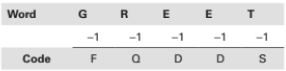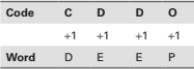# Mathematical Reasoning - Coding Decoding

>>>>>>>>Coding Decoding

• A

BTEAE• B

BTAEA• C

BATEA• D

None of the above• Option : A
• Explanation : The coding is done as follows:Accordingly, the code for LINEN is BTEAE.

• A

DEEPS• B

DEESP• C

DESEP• D

SPEDE• Option : A
• Explanation : By careful observation, we can say that the letters have been written in the reverse order. Hence, SPEED will be written DEEPS; therefore, (a) is the answer.

• A

EDDP• B

DEEP• C

PEED• D

EPED• Option : B
• Explanation : Here, it is −1 for each letter of the word ‘GREET’.CDDO itself is a code. To find out what it stands for, we have to add +1 to the alphabets to get back the original word.• A

HISTBCDEFG• B

HISTBCDEGF• C

HISBTCDEFG• D

HISTBCEDFG• Option : A
• Explanation : The pattern of coding is such that each letter has been allotted the value of 2 letters following the sequence, i.e., A = BC, B = CD, C = DE, etc. Hence, the word GRACE will be coded as ‘HISTBCDEFG’. Therefore, option (a) is the answer.

• A

LEEF• B

FEEL• C

DEAL• D

REEL• Option : B
• Explanation : Refer to alphabet series in Table 5.1 starting from the L.H.S. pair, E is the antecedent of F and G is the precedent of F. We get EG as code for F; HJ as code for I; and so on.
Similarly, EGDFDFKM is the code for FEEL. F is coded as EG with its precedent and antecedent letters. Similarly, ‘I’ is coded as HJ and L as KM. Hence, EGDFDFKM stands for FEEL. Therefore, option (b) is the correct answer.

## Description

• Coding And Decoding Questions can be used to give quizzes by any candidate who is preparing for UGC NET Paper 1
• This Coding And Decoding Questions section will help you test your analytical skills in a tricky method, thereby giving you an edge over other students
• All candidates who have to appear for the Kendriya Vidyalaya Entrance exam can also refer to this mcq section.
• You can also get access to the Coding And Decoding MCQ ebook.
• Coding And Decoding Questions can be used in the preparation of JRF, CSIR, and various other exams.